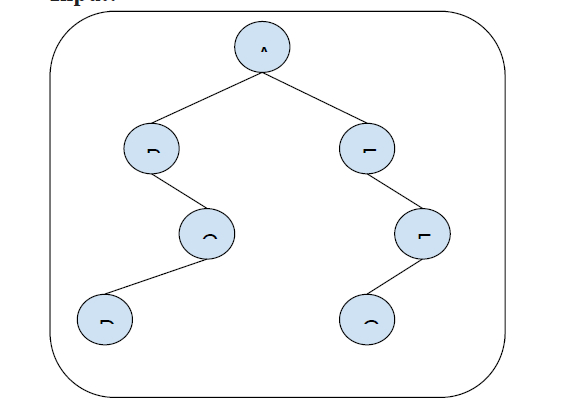# Find mirror of a given node in Binary tree in C++

In this problem, we are given a binary tree. Our task is to Find mirror of a given node in the Binary tree. We will be given a node, and find the mirror image of that node in the opposite subtree.

Let’s take an example to understand the problem,

## Input## Output

mirror of B is E.

## Solution Approach

One simple solution to solve the problem is by using the recursion from the root using two pointers for left subtree and right subtree. Then for the target value if any mirror is found return the mirror otherwise recur other nodes.

Program to illustrate the working of our solution,

## Example

Live Demo

#include <bits/stdc++.h>
using namespace std;
struct Node {
int key;
struct Node* left, *right;
};
struct Node* newNode(int key){
struct Node* n = (struct Node*) malloc(sizeof(struct Node*));
if (n != NULL){
n->key = key;
n->left = NULL;
n->right = NULL;
return n;
}
else{
cout << "Memory allocation failed!"
<< endl;
exit(1);
}
}
int mirrorNodeRecur(int node, struct Node* left, struct Node* right){
if (left == NULL || right == NULL)
return 0;
if (left->key == node)
return right->key;
if (right->key == node)
return left->key;
int mirrorNode = mirrorNodeRecur(node, left->left, right->right);
if (mirrorNode)
return mirrorNode;
mirrorNodeRecur(node, left->right, right->left);
}
int findMirrorNodeBT(struct Node* root, int node) {
if (root == NULL)
return 0;
if (root->key == node)
return node;
return mirrorNodeRecur(node, root->left, root->right);
}
int main() {
struct Node* root = newNode(1);
root-> left = newNode(2);
root->left->left = newNode(3);
root->left->left->left = newNode(4);
root->left->left->right = newNode(5);
root->right = newNode(6);
root->right->left = newNode(7);
root->right->right = newNode(8);
int node = root->left->key;
int mirrorNode = findMirrorNodeBT(root, node);
cout<<"The node is root->left, value : "<<node<<endl;
if (mirrorNode)
cout<<"The Mirror of Node "<<node<<" in the binary tree is
Node "<<mirrorNode;
else
cout<<"The Mirror of Node "<<node<<" in the binary tree is
not present!";
node = root->left->left->right->key;
mirrorNode = findMirrorNodeBT(root, node);
cout<<"\n\nThe node is root->left->left->right, value :
"<<node<<endl;
if (mirrorNode)
cout<<"The Mirror of Node "<<node<<" in the binary tree is
Node "<<mirrorNode;
else
cout<<"The Mirror of Node "<<node<<" in the binary tree is
not present!";
}

## Output

The node is root->left, value : 2
The Mirror of Node 2 in the binary tree is Node 6

The node is root->left->left->right, value : 5
The Mirror of Node 5 in the binary tree is not present!